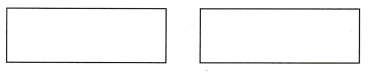# McGraw Hill Math Grade 2 Chapter 7 Lesson 9 Answer Key Equal Parts With Different Shapes

Practice questions available in McGraw Hill Math Grade 2 Answer Key PDF Chapter 7 Lesson 9 Equal Parts With Different Shapes will engage students and is a great way of informal assessment.

## McGraw-Hill Math Grade 2 Answer Key Chapter 7 Lesson 9 Equal Parts With Different Shapes

Solve

Question 1.
Which shapes have one half colored red? Circle them.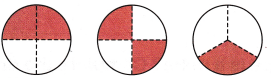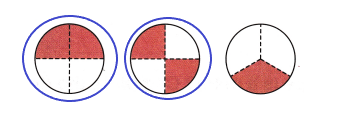Explanation:
I drew circles around the first and second shape
They are colored one half red.

Draw a line in each shape. Show two ways to make halves.

Question 2.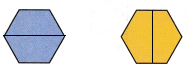Explanation:
I drew a line in each shape to show two ways to make halves.

Question 3.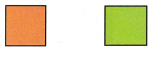Explanation:
I drew a line in each shape to show two ways to make halves.

Question 4.
Show two ways to make thirds. Color a third of each shape red.